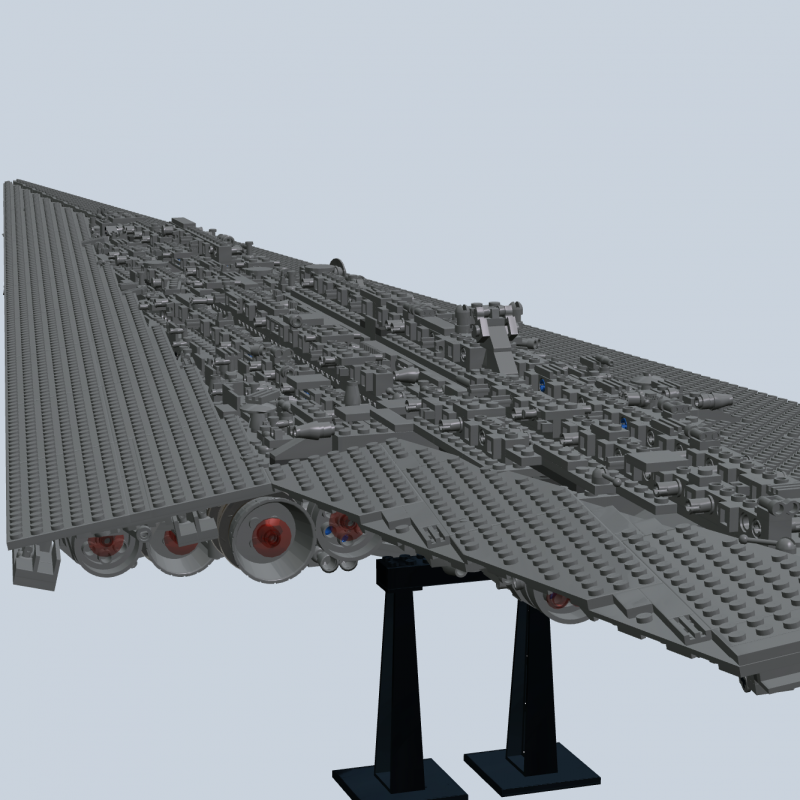The adventures of building a web renderer

RE: The adventures of building a web renderer
#7
Merging points efficiently

From my post regarding indexing, you could see how using 'indexes' could help reduce the amount of points.

As an example. Consider a 3D box. I has 8 corners. All lines and triangles use these 8 corners, but a box is constructed by 12 lines and 12 triangles. Each line has 2 points and each triangle has 3. With each point taking 3 numbers, the amount of numbers stored to show a box is:

(12*2 + 12*3)*3 = 180 numbers.

If we store the 8 corner points separately (8*3 = 24 numbers) and simply store offsets/indices, the "*3" from the previous equation can be removed, resulting in:

24 + (12*2 + 12*3) = 84 numbers.

Parts in the LDraw library (especially standard parts) have a lot of common points, so it makes sense to use this trick to save memory, and thereby also rendering time. In our example above we save roughly 50%, so let us take a look at how much we can save in our test model.

I would also like to introduce you to an additional test model. This is the very first LDraw model I ever built. It is quite big (3500+ parts) and is good for stress testing:Here are the baseline numbers for just showing triangles and not using our trick to combine points. I call the two models 'Psych' (the blue car) and 'Executor':

Psych: Memory usage: 36.3MB. Rendering time: 1.039ms. Number of points: 375.432.
Executor: Memory usage: 862MB. Rendering time: 1.8185ms. Number of points: 11.333.253.

This is what happens when you combine points for the full models:

Psych: Memory usage: 16.6MBRendering time: 3.121ms. Number of points: 99.687.

Executor: Memory usage: 313MBRendering time: 99.495ms. Number of points: 2.700.145.

That rendering time is completely unacceptable. Here is what happens when points are only combined for the individual parts (not the full mode:

Psych: Memory usage: 20.5MBRendering time: 1.584ms. Number of points: 100.339.

Executor: Memory usage: 414MBRendering time: 17.096ms. Number of points: 2.751.714.

These tradeoffs are much more acceptable. Next up was adding normal lines to the mix.
« Next Oldest | Next Newest »